Attachments are accessible only for community members.
Log in# PSoC5LP: Sending LED Color data via PWM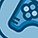Level 6
Level 6Hello!

I'm working on implementing a protocol that utilizes PWM to do 1 wire transmission to LTST-E263CEGBK RGB LEDs and having some trouble getting it working.

I'm using a CY8CKIT-050.

The 5th page of the datasheet describes the protocol: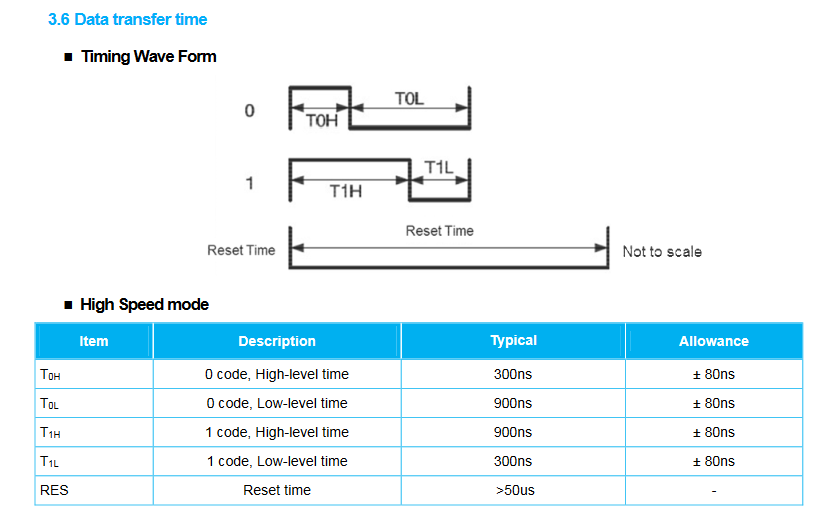Each color is communicated by 8 bits (periods), transmitted in sequence, for a total of 24, and capped off by a low time of at least 50uS.  According to this datasheet I need a 800KHz PWM to meet the timing spec.

I figured I could tie the PWM to the clock input of a counter to determine when 24 periods have elapsed, and send a pulse (~52uS using a 19KHz out_clk) to reset the PWM component as well as the counter when a match occurs.  My PSoC hardware is laid out as follows: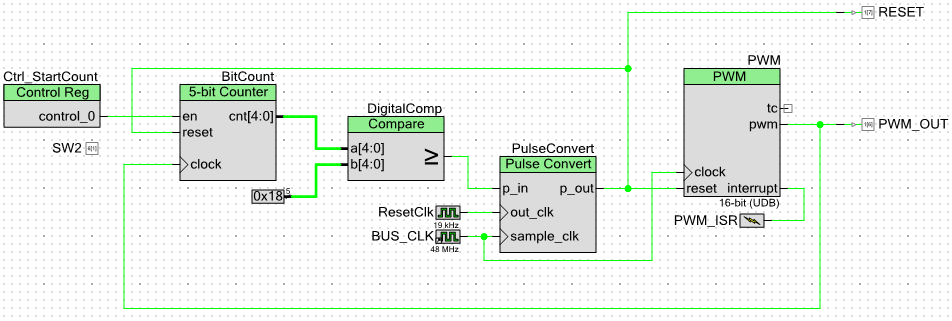For whatever reason (probably missing something obvious) I can't get this to work.  The code is rather simple:

`#define PWM_VAL_0   14#define PWM_VAL_1   42#define MAX_BIT_INDEX   8#define G_INDEX 0#define R_INDEX 1#define B_INDEX 2static volatile uint8_t Bytes_Out = {0x00, 0xFF, 0xAA};void PWM_ISR_Interrupt_InterruptCallback(void){    static uint8_t byte_index = 0;    static uint8_t bit_index = MAX_BIT_INDEX;    PWM_ReadStatusRegister();        if (Ctrl_StartCount_Read() > 0){                if (((Bytes_Out[byte_index] >> --bit_index) & 0x01) > 0){            PWM_WriteCompare(PWM_VAL_1);        } else {            PWM_WriteCompare(PWM_VAL_0);        }                if (bit_index == 0){            byte_index++;            bit_index = MAX_BIT_INDEX;        }                if (byte_index >= 3){            byte_index = 0;               Ctrl_StartCount_Write(0);        }        } else {        PWM_WriteCompare(PWM_INIT_COMPARE_VALUE1);    }        PWM_ISR_ClearPending();}int main(){    CyGlobalIntEnable; /* Enable global interrupts. */        PWM_Start();    PWM_ISR_Start();        for(;;)    {        if (SW2_Read() == 0 && Ctrl_StartCount_Read() == 0){            Ctrl_StartCount_Write(1);        }            }}`

Should be a simple write to my StartCount register that should enable the counter, then the next call to the PWM TC Interrupt will begin generating pulses and increment the counter, and the PWM will be reset at the end of 24 PWM Periods.

What I'm finding is that Ctrl_StartCount_Read() is never returning 1, even after a write a 1 to it when pressing SW2.  I'm hitting the PWM ISR without a problem, but I never drop in to if (Ctrl_StartCount_Read() > 0).

I have attached the project.  Would anybody be able to call out where I'm going wrong here?  I don't understand why after writing a 1 to Ctrl_StartCount it reads back inside the ISR as 0.  I have attached the project if anyone out there with a CY8CKIT-050 could take a look it would be greatly appreciated.

Thanks!

1 SolutionAttachments are accessible only for community members.
Log in# Re: PSoC5LP: Sending LED Color data via PWMLevel 9
Level 9
Distributor - Marubun (Japan)Hi,

Your design is too difficult for me to understand.

So I tried (for me) simpler approach using verilog.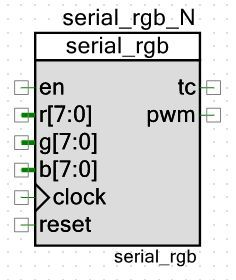verilog source

======================

`include "cypress.v"

//`#end` -- edit above this line, do not edit this line

//`#start body` -- edit after this line, do not edit this line

module serial_rgb(

input        en,

input  [7:0] r,

input  [7:0] g,

input  [7:0] b,

input        clock,

input        reset,

output reg   tc,

output reg   pwm

) ;

reg[6:0] count ; /* count[6:5] RGB, count[4:2] bit pos, count[1:0] pwm pos */

function pwm_gen;

input value ;

input [1:0] pos ;

begin

case (pos)

2'd0: pwm_gen = 1'b1 ;

2'd1: pwm_gen = (value == 1'b1) ? 1'b1 : 1'b0 ;

2'd2: pwm_gen = (value == 1'b1) ? 1'b1 : 1'b0 ;

2'd3: pwm_gen = 1'b0 ;

endcase

end

endfunction

always @ (posedge reset or posedge clock) begin

if (reset == 1'b1) begin

count      <= 7'd0 ;

pwm        <= 1'b0 ;

end else if (en == 1'b1) begin

if (count == 7'b1011111) begin

tc <= 1'b1 ;

count <= 7'd0 ;

end else begin

tc <= 1'b0 ;

count <= count + 7'd1 ;

end

case (count[6:5])

2'd0:    pwm <= pwm_gen(r >> count[4:2], count[1:0]) ;

2'd1:    pwm <= pwm_gen(g >> count[4:2], count[1:0]) ;

2'd2:    pwm <= pwm_gen(b >> count[4:2], count[1:0]) ;

default: pwm <= 1'b0 ;

endcase

end

end

endmodule /* serial_rgb */

======================

Then schematic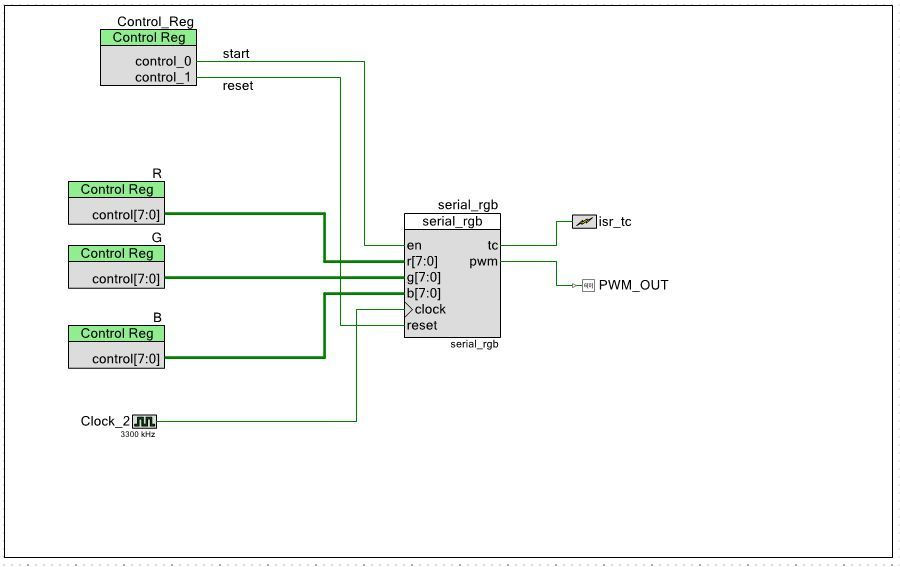pin assign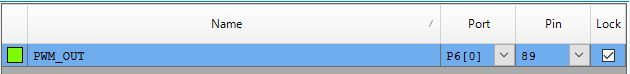main.c

=========================

#include <project.h>

#define BIT_CLEAR 0x00

#define BIT_ENABLE 0x01

#define BIT_RESET 0x02

volatile int data_sent = 0 ;

CY_ISR(tc_isr)

{

isr_tc_ClearPending() ;

data_sent = 1 ;

}

int main()

{

isr_tc_ClearPending() ;

isr_tc_StartEx(tc_isr) ;

CyGlobalIntEnable;

Control_Reg_Write(BIT_RESET) ; /* reset */

Control_Reg_Write(BIT_CLEAR) ;

R_Write(0x00) ;

G_Write(0xFF) ;

B_Write(0xAA) ;

Control_Reg_Write(BIT_ENABLE) ;

for(;;)

{

if (data_sent) {

data_sent = 0 ;

}

}

}

=========================

As far as seeing the wave from via Oscilloscope the '0' patterns and '1' patterns were generated.

But as I don't have the device, I'm not sure if this works for your target device.

So please proceed with a grain of salt 😉

moto

4 RepliesAttachments are accessible only for community members.
Log in# Re: PSoC5LP: Sending LED Color data via PWMLevel 9
Level 9
Distributor - Marubun (Japan)Hi,

Your design is too difficult for me to understand.

So I tried (for me) simpler approach using verilog.verilog source

======================

`include "cypress.v"

//`#end` -- edit above this line, do not edit this line

//`#start body` -- edit after this line, do not edit this line

module serial_rgb(

input        en,

input  [7:0] r,

input  [7:0] g,

input  [7:0] b,

input        clock,

input        reset,

output reg   tc,

output reg   pwm

) ;

reg[6:0] count ; /* count[6:5] RGB, count[4:2] bit pos, count[1:0] pwm pos */

function pwm_gen;

input value ;

input [1:0] pos ;

begin

case (pos)

2'd0: pwm_gen = 1'b1 ;

2'd1: pwm_gen = (value == 1'b1) ? 1'b1 : 1'b0 ;

2'd2: pwm_gen = (value == 1'b1) ? 1'b1 : 1'b0 ;

2'd3: pwm_gen = 1'b0 ;

endcase

end

endfunction

always @ (posedge reset or posedge clock) begin

if (reset == 1'b1) begin

count      <= 7'd0 ;

pwm        <= 1'b0 ;

end else if (en == 1'b1) begin

if (count == 7'b1011111) begin

tc <= 1'b1 ;

count <= 7'd0 ;

end else begin

tc <= 1'b0 ;

count <= count + 7'd1 ;

end

case (count[6:5])

2'd0:    pwm <= pwm_gen(r >> count[4:2], count[1:0]) ;

2'd1:    pwm <= pwm_gen(g >> count[4:2], count[1:0]) ;

2'd2:    pwm <= pwm_gen(b >> count[4:2], count[1:0]) ;

default: pwm <= 1'b0 ;

endcase

end

end

endmodule /* serial_rgb */

======================

Then schematicpin assignmain.c

=========================

#include <project.h>

#define BIT_CLEAR 0x00

#define BIT_ENABLE 0x01

#define BIT_RESET 0x02

volatile int data_sent = 0 ;

CY_ISR(tc_isr)

{

isr_tc_ClearPending() ;

data_sent = 1 ;

}

int main()

{

isr_tc_ClearPending() ;

isr_tc_StartEx(tc_isr) ;

CyGlobalIntEnable;

Control_Reg_Write(BIT_RESET) ; /* reset */

Control_Reg_Write(BIT_CLEAR) ;

R_Write(0x00) ;

G_Write(0xFF) ;

B_Write(0xAA) ;

Control_Reg_Write(BIT_ENABLE) ;

for(;;)

{

if (data_sent) {

data_sent = 0 ;

}

}

}

=========================

As far as seeing the wave from via Oscilloscope the '0' patterns and '1' patterns were generated.

But as I don't have the device, I'm not sure if this works for your target device.

So please proceed with a grain of salt 😉

moto

# Re: PSoC5LP: Sending LED Color data via PWMLevel 6
Level 6moto,

Very cool, I will give that a try!

# Re: PSoC5LP: Sending LED Color data via PWMLevel 6
Level 6moto,

I've been playing around with your component today, it mostly works as I need it to, but there were a couple tweaks:

First was byte order (which I forgot to specify, oops!).  I need bytes to go out MSb to LSb.  So I adjusted the calls to pwm_gen:

case (count[6:5])

2'd0:    pwm <= pwm_gen(g >> (3'd7 - count[4:2]), count[1:0]);

2'd1:    pwm <= pwm_gen(r >> (3'd7 - count[4:2]), count[1:0]);

2'd2:    pwm <= pwm_gen(b >> (3'd7 - count[4:2]), count[1:0]);

default: pwm <= 1'b0;

endcase

I was also seeing an extra period go out at the end of the transmission.  A little adjustment of the counter sorted that out:

if (count == 7'b1011011) begin

tc <= 1'b1;

count <= 7'd0;

end else begin

tc <= 1'b0;

count <= count + 7'd1;

end

I am a little bit confused as to why 95 (0b1011111) was wrong (8 Bytes * 3 Transmissions = 96 => 95) but reducing the count by 1 period => 91 works and now I've got the LEDs lighting up in proper color.  I appreciate the help, this likely saved me a whole bunch of time.

This was the first time I had seen any HDL code in a very long time (since university I think), so it was definitely a refresher.

Thanks again!

# Re: PSoC5LP: Sending LED Color data via PWMLevel 9
Level 9
Distributor - Marubun (Japan)Hi,

> I've been playing around with your component today, it mostly works as I need it to, but there were a couple tweaks:

Yes, it's the place you need "real" device to test.

Although I was anxious if you were not familiar with HDLs, but I'm glad that you are.

About the MSB first or LSB first,

it's a pretty popular symptom to be debugged,

and you've already fixed it. (thanks)

For the off-by-one counter,

may be register variables being used affect the timing

or may be there is/are other reason(s),

but

> and now I've got the LEDs lighting up in proper color.

This is the most important bottom line 😉

> I appreciate the help, this likely saved me a whole bunch of time.

This line is a great reward for me, thank you, too!

moto